Latest Banking jobs   »

# Quantitative Aptitude Quiz For SBI Clerk Prelims 2022- 19th May

Q1. Find in how many ways the word ‘INTICINCO’ can be written so that ‘T’ always comes at end?
(a) 720
(b) 1680
(c) 5040
(d) 1024
(e) 210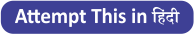Q2. Find the probability of selecting a two-digit number at random such that it should be a multiple of 3 but not a multiple of 12?
(a) 4/15
(b) 23/90
(c) 7/30
(d) 1/3
(e) 11/45

Q3. A rectangular field was fenced at 4 Rs./meter and ratio of breadth to length of field is 9 : 4. If total cost of fencing was Rs. 208, then find area of the rectangular field?
(a) 144m²
(b) 72m²
(c) 180m²
(d) 108m²
(e)36m²

Q4. A bag contains 10 two-rupee coins, 5 ten-rupee coins and 7 one-rupee coins, if 3 Coins are withdrawn at random, then find the probability of getting minimum amount?
(a) 1/50
(b) 1/22
(c) 1/66
(d) 1/44
(e) 1/33

Q5. If the diameter of a wheel is 46 cm, then how many revolutions it will take to cover 1012 meter?
(a) 550 revolutions
(b) 800 revolutions
(c) 700 revolutions
(d) 600 revolutions
(e) 750 revolutions

Q6. If three dice are thrown simultaneously, then find the probability of getting a sum of 17?
(a) 1/72
(b) 1/54
(c) 1/45
(d) 1/36
(e) None of these

Q7. If ratio of area of rectangle to its perimeter is 9 : 2 and breadth of rectangle is 33⅓% of it’s length, then find area of rectangle?
(a) 400unit²
(b) 240unit²
(c) 364unit²
(d) 432unit²
(e) can’t be determined

Q8. Ankit speaks truth 3 out of 5 times and Pankaj speaks truth 2 out of 3 times. Find the probability of that they contradict one other?
(a) 4/15
(b) 1/3
(c) 1/6
(d) 7/15
(e) None of these

Q9. The total surface area of a right circular cylinder having base radius ‘R’ and height ‘h’ is obtained by dividing its volume by what expression?
(a) R/4
(b) Rh/2(R+h)
(c) R/2
(d) (R+h)/2Rh
(e) Rh/4(R+h)

Q10. A basket contains 6 Red balls and 9 Green balls while another basket contains 7 Red balls and 8 Green balls. If one ball is drawn from either of the two baskets, then what is the probability of drawing a green ball?
(a) 7/30
(b) 8/15
(c) 17/30
(d) 11/15
(e) 13/30

Q11. If two dice are thrown simultaneously, then find the probability of getting a sum of 9?
(a) 1/6
(b) 2/3
(c) 1/15
(d) 1/9
(e) 1/18

Q12. The curved surface area and total surface area of right circular cylinder are in the ratio of 2 :3. If the total surface area of the cylinder is 3696 cm², then find the volume of the cylinder?
(a) 14312 cm³
(b) 16242 cm³
(c) 14644 cm³
(d) 17248 cm³
(e) 19286 cm³

Q13. Three are 5 green balls, 7 blue balls and 3 red balls in a bag. If 2 balls are chosen randomly from the bag, then find the probability that at least 1 ball is green ball?
(a) 1/9
(b) 2/7
(c) 3/8
(d) 3/5
(e) 4/7

Q14. If three coins are tossed simultaneously, then find the probability of obtaining exactly 2 heads?
(a) 1/8
(b) 3/8
(c) 1/4
(d) 3/4
(e) None of the above.

Q15. If length of rectangle A is 1.25 times of its width and area of rectangle A is 1280 cm². If width of rectangle A is equal to side of equilateral triangle, then find the perimeter of triangle?
(a) 72m
(b) 66m
(c) 84m
(d) 96m
(e) 60m

Solutions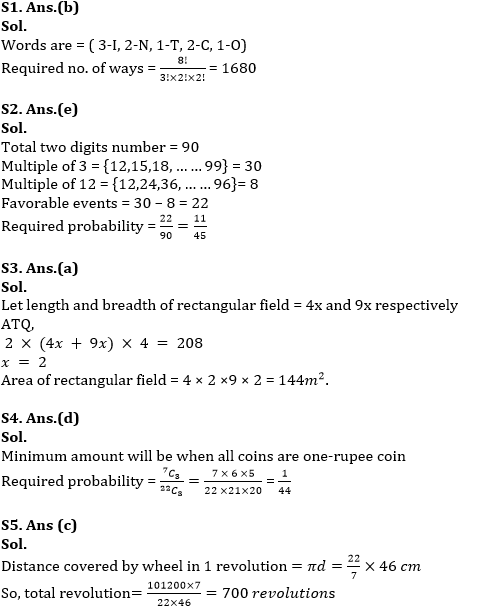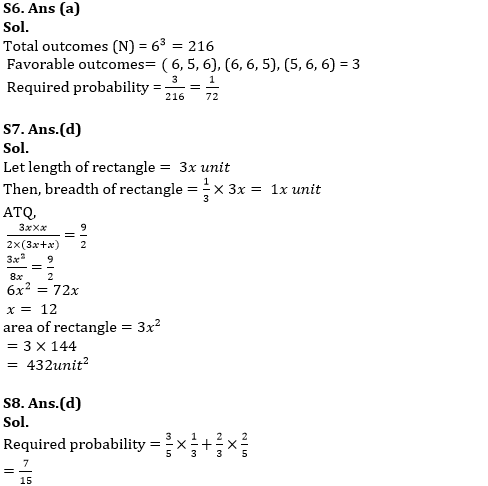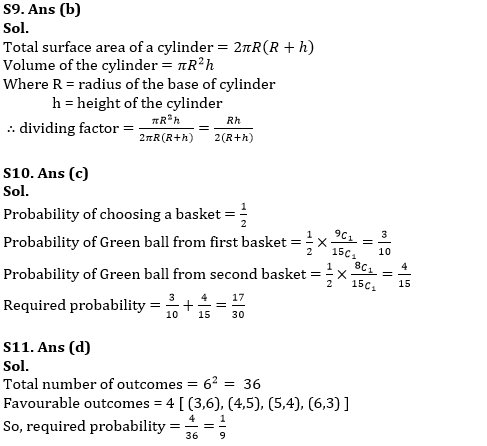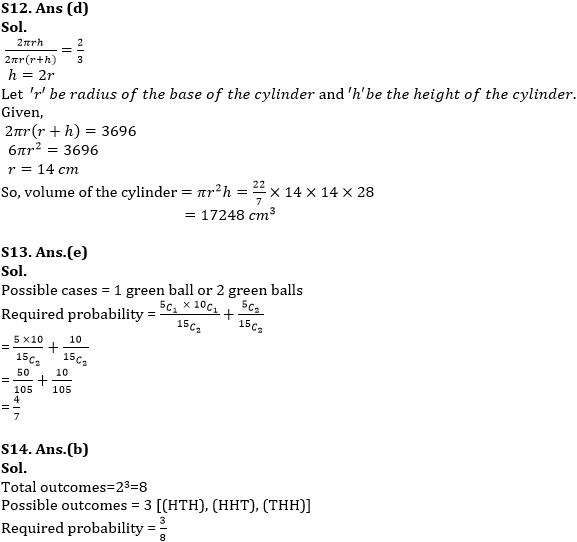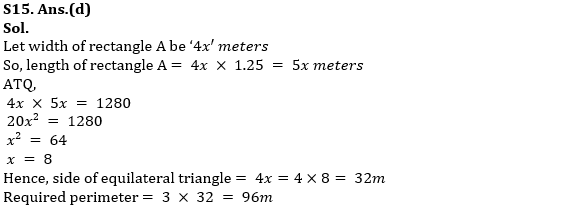#### Congratulations!